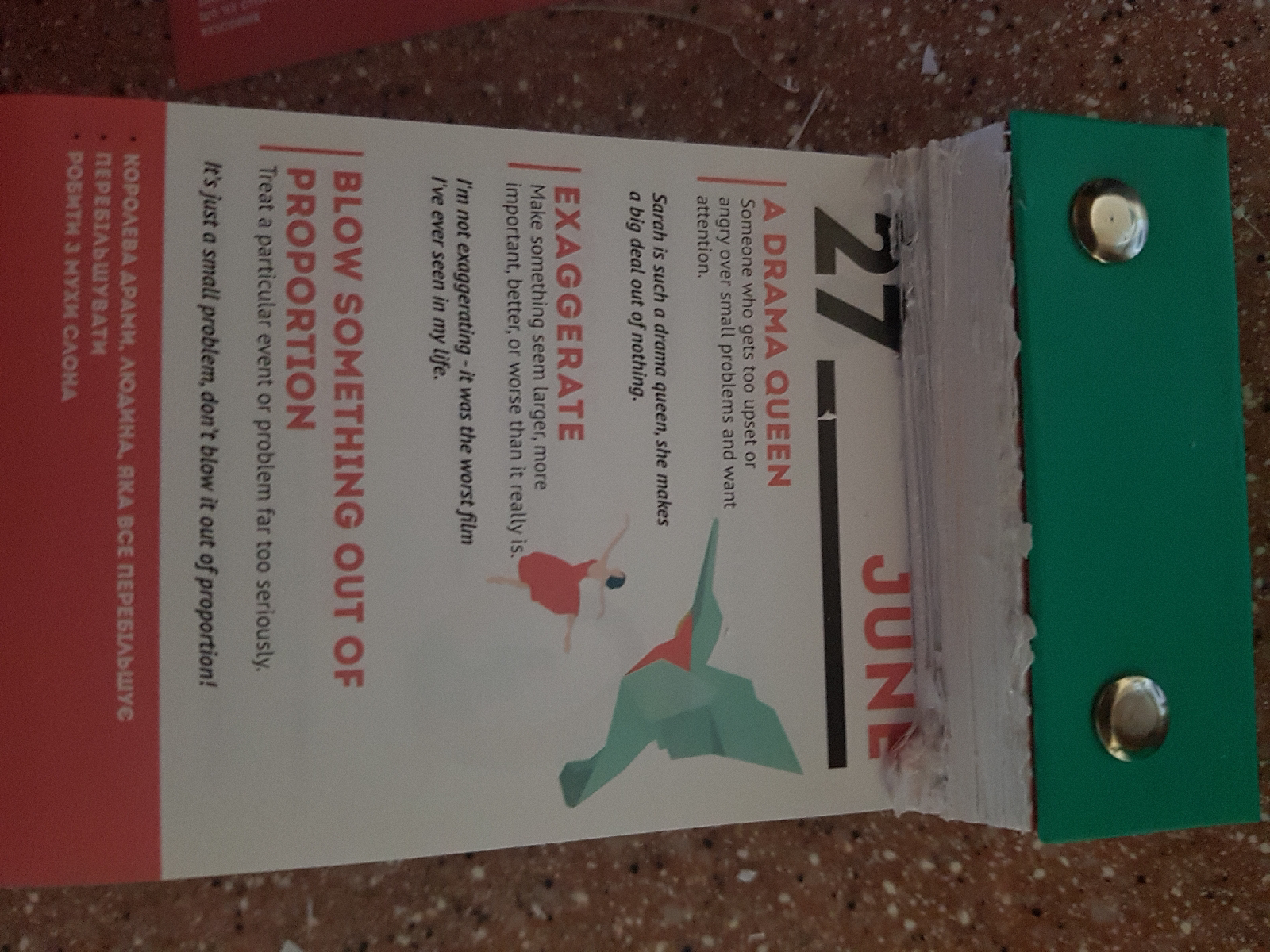# Fun Holiday – Fibonacci Day

November 23 is Fibonacci Day, an annual holiday that honors one of the most influential mathematicians of the Middle Ages - Leonardo Bonacci.

Also known as Leonardo of Pisa, he is popularly known as Leonardo Fibonacci. Fibonacci is a contraction of filo Bonacci, meaning the son of Bonaccio.
Date Sequence
November 23 is celebrated as Fibonacci day because when the date is written in the mm/dd format (11/23), the digits in the date form a Fibonacci sequence: 1,1,2,3. A Fibonacci sequence is a series of numbers where a number is the sum of the two numbers before it. For example: 1, 1, 2, 3...is a Fibonacci sequence. Here, 2 is the sum of the two numbers before it (1+1). Similarly, 3 is the sum of the two numbers before it (1+2).
The Rabbit Question
The Fibonacci sequence, one of the biggest accomplishments of Leonardo of Pisa came from a simple puzzle about rabbit population. In his book Liber acaci, Fibonacci posed this puzzle: if there are a pair of newly born rabbit - male and female - in the field and if they are able to produce another pair of rabbits in their second month of life, how many pairs of rabbits will be there after a year?
Born in 1170 in Pisa, Italy, Fibonacci was also responsible for making the Hindu-Arabic numerals popular in Europe. In Liber acaci he advocated the use of these numerals, explained the use of zero, provided ways to convert between currencies and different measurements, and described how to calculate interest.
Fibonacci in Nature
One of the beauties of the Fibonacci sequence is that the series is evident all over the natural world. Petal arrangements in flowers, the ordering of leaves in plants, the shell of the nautilus, the DNA molecule and even hurricanes show patterns that correspond to the sequence.
How to Celebrate?
• Start the day by learning more about the Fibonacci sequence and its theoretical and practical uses.
• A number of fruits and vegetables, like pineapples, romanesco (a cross between broccoli and cauliflower) display the Fibonacci series - incorporate them in your meals to celebrate this mathematical holiday.
• Have children in your life? Introduce them to the elegance of math and the importance of learning it to use in real life.
Did You Know…
…that the ratio of two successive numbers in the Fibonacci sequence is very close to the Golden Ratio? The Golden Ratio is approximately equal to 1.6. Objects whose length and breadth exhibit the Golden Ratio are thought to be the most pleasing to the eye.